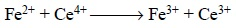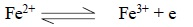Home | | Pharmaceutical Drug Analysis | Volumetric Analysis

# Volumetric Analysis

Volumetric analysis may be broadly defined as those analytical methods whereby the exact volume of a solution of known concentration actually consumed during the course of an analysis is considered as a measure of the amount of active constituent in a given sample under determination (assay).

VOLUMETRIC ANALYSIS

Volumetric analysis may be broadly defined as those analytical methods whereby the exact volume of a solution of known concentration actually consumed during the course of an analysis is considered as a measure of the amount of active constituent in a given sample under determination (assay).

## 1. THEORY

According to the official method of analysis, hydrochloric acid can be determined by first weighing a given sample accurately, and secondly, by adding carefully a solution of known strength of sodium hydroxide in the presence of an appropriate indicator unless and until the exact equivalent amounts of HCl and NaOH have undergone the following chemical reaction :Analyte (or Active Constituent) is the chemical entity under assay e.g., HCl.

Titrant is the solution of known strength (or concentration) employed in the assay e.g., NaOH.

Titration is the process of adding and then actually measuring the volume of titrant consumed in the assay. This volume is usually measured by the help of a calibrated burette.

Indicator is a chemical substance sensitive enough to display an apparent change in colour very close to the point in the ongoing titration process at which equivalent quantities of analyte and titrant have almost virtually reacted with each other.

Equivalence Point (or Stoichiometric Point) is the point at which there appears an abrupt change in certain characteristic of the prevailing reaction mixture—a change that is either ascertained electrometrically or is visibly spotted by the use of indicators.

In usual practice, the volumetric titrations may be accomplished either by direct titration method e.g., assay of HCl employing NaOH as the titrant, or by residual titration method e.g., assay of ZnO in which case a known-excess-measured volume of standardised solution of H2SO4, more than the actual amount chemically equivalent to ZnO, is added to the sample ; thereupon, the H2SO4 which remain unreacted with ZnO is subsequently titrated (sometimes referred to as back titration or residual titration in the text) employing standardized NaOH solution.

Thus, we have :

Known amount of H2SO4 consumed Known amount of NaOH + Unknown amount of ZnO Most official compendia usually record the results of drug assays in terms of % w/v, % w/w and % v/v.

## 2. DEFINITIONS

In order to have a clear-cut understanding of the various calculations involving volumetric assays through-out this book one needs to gain an in-depth knowledge of the various terms related to ‘equivalents’. They are :

(a) Gram-equivalent Weight (GEW) : It is the weight in grams that is chemically equivalent to 1 gram-atom of hydrogen (1.0079 g).

It is also sometimes simply referred to as the ‘gram-equivalent’. However, GEW has two distinct definitions for neutralization as well as as oxidation-reduction reactions as stated below :

(i)          For Neutralization Reactions : GEW is defined as that weight of a substance in grams which contains, furnishes, reacts directly or indirectly and replaces 1 gram-atom or ion of hydrogen.

(ii) For Oxidation—Reduction Reactions

Explanation : A reaction usually takes place by the combination of oxidizing and reducing agents and this may be considered as the basis for the quantitative measurement of one of the reactants. For instance, FeSO4 can be determined quantitatively by its reaction with ceric sulphate [Ce(SO4)2] as expressed by the following equation :.......(a)

Equation (a) can be split into two half-equations as shown below thereby depicting the loss of elec-trons by the Fe2+ ion [Eq. (b)] and the gain of electrons by the Ce4+ [Eq. (c)] :...........(b).................(c)

From Eq. (a) it is evident that each molecule of FeSO4, upon oxidation, happens to lose one electron. Hence, one mole of FeSO4 loses 6.02 × 10 23 electrons which is equivalent to 1 Faraday or 96,500 C. Thus, in electrochemical determination of equivalence point the quantity of electricity is almost identical with that required to reduce 1 mole of Ce(SO4)2. It follows from here that 1 mole of FeSO4 and 1 mole of Ce(SO4)2 are chemical equivalents. In other words, 1 g of H, acting as a reducing agent, loses electrons equivalent to 96,500 C.

(b) Equivalent Weight of a Reducing Agent is that weight which loses electrons equivalent to 96,500 C.

It may be calculated by dividing the gram-molecular weight by the number of electrons lost by each molecule, for instance :hence, the equivalent weight of FeSO4 oxidizing to Fe2(SO4)3 comes out to be 151.919 [FeSO4 :

molecular weight = 151.91] or 1 gram-molecular weight.

(c) Equivalent Weight of an Oxidizing Agent is that weight which gains electrons equivalent to 1 Faraday, or to the electrons gained by 1 gram-ion of H+ ions (2H+ + 2e H2 ).

It may be calculated by dividing the gram-molecular weight by the number of electrons gained by each molecule, for example :

(a)   Ce4+ + e   → Ce3+      (cerous ion)

hence, the equivalent weight of ceric sulphate is 1 gram-molecular weight 332.24 g [Ce(SO4)2 : molecular weight = 332.24]

(b) MnO4– + 5e  → Mn2+   (manganous ion)

hence, the equivalent weight of potassium permanganate is 1/5th gram-molecular weight 31.61 g.

(KMnO4 : 1/5 × 158.05 = 31.61)

(c)   Cr2O72– + 6e —  2Cr3+                    (chromous ion)

hence, the equivalent weight of potassium dichromate is l/6 gram-molecular weight 49.03 g. (K2Cr2O7 : 1/6 × 294.18 = 49.03)

(d) I2 + 2e  → 2I–  (iodide ion)

hence, the equivalent weight of iodine is 1 gram-molecular weight 126.90 g. (I2 : Molecular Weight = 126.90)

(e)    BrO3 + 6e   → Br                            (bromide ion)

hence, the equivalent weight of potassium bromate is 1/6 gram-molecular weight 27.83 g.

(KBrO3 : 1/6 × 167.01 = 27.83)

(d) Gram-milliequivalent Weight (GmEW) is nothing but GEW/1000. This term is very much used in all types of volumetric calculations.

(e) Equivalent (equiv) is the number of gram-equivalents involved in a quantitative method.

(f) Milliequivalent (meq) is the number of gram-milliequivalents involved in a quantitative method.

However, meq is used more frequently than equiv in quantitative procedures.

(g) Standard Solution is a solution of known (pre-determined) normality or molarity.

(h) Normality (expression of concentration) is the number of equivalents of solute per litre (equiv/lire) or milliequivalents per ml. (meg/ml) solution.

(i) Molarity is the expression of the concentration of a solution in terms of moles per litre.

(j) Standardization is the actual determination of either the normality or the molarity of a solution.

(k) Primary Standard is the substance of known purity (‘AnalaR’-grade reagents) whose carefully weighed quantity helps in the standardization of an unknown solution (normality or molarity).

(l) Secondary Standard is another standard solution that is used for standardization of an unknown solution.

Example : An unknown solution of HCl may be standardized volumetrically in two ways, namely :

(i) by the help of ‘AnalaR’-grade Na2CO3 i.e., purity is known-‘Primary Standard’, and

(ii) by the help of another standard solution of NaOH—‘Secondary Standard’.

(m) Titer : is the weight of a substance chemically equivalent to 1 ml of a standard solution.

Example : 1 ml of 1 N HCl contains 0.03646 g (i.e., 0.001 equiv or 1 meq) of HCl and hence is chemically equivalent to 0.04000 g (i.e., 0.001 equiv or 1 meq) of NaOH.

Thus, most calculations in volumetric determinations (titrimetry) are enormously facilitated by using titer values.

For instance, in the offcial procedure for the assay of tartaric acid, it is stated that ‘Each millilitre of 1 N sodium hydroxide is equivalent to 75.04 mg of C4H6O6’. The C4H6O6 titer of 1 N sodium hydroxide is, therefore, 75.04 mg/ml, a value that may be calculated as follows :

An examination of the equation indicates that 1 mole or 150.09 g ofis 2 equiv, and the equivalent weight of H2C4H4O6 is 75.04 g. Hence, each millilitre of 1 NaOH contains 0.001 equiv of NaOH and is equivalent to 0.001 equiv or 0.001 × 75.04 = 0.07504 g or 75.04 mg of H6C4O6.

Study Material, Lecturing Notes, Assignment, Reference, Wiki description explanation, brief detail
Pharmaceutical Drug Analysis: Theory and Technique of Quantitative Analysis : Volumetric Analysis |